##### Search### SECTION A (25 Marks)

Answer all the questions in this section in the spaces provided.
1.

Degree of accuracy ± 0.1 cm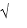1 marks

2.

a)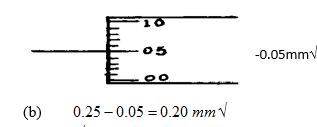2 marks

3.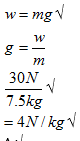3 marks

4.

A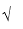Pressure in liquids increases with depth so wall should be thicker as depth increases.2 marks

5.

Brass expands more than ironand so on heating the strip bends upwards establishing constantcurrent flows and the siren goes off3 marks

6.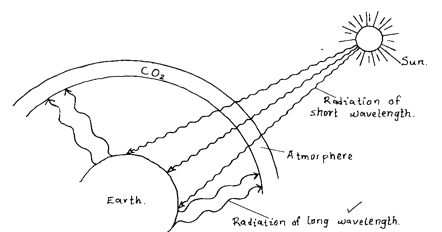CO2 layer allows short wave radiation from the sun to pass easily to the surface of the earth but does not allow the longer wanelength of radiation from the earth’s surface to pass through.3 marks

7.

The particles of sugar are spread out evenly in the water, as the water particle increases the particles of sugar are further spread and this makes the sugar taste less and less.

1 marks

8.

Sum clockwise moment = sum of anticlockwise moments.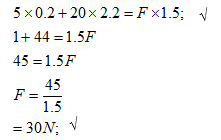2 marks

9.

Stable equilibrium. When the block is displaced it goes back to the original position

3 marks

10.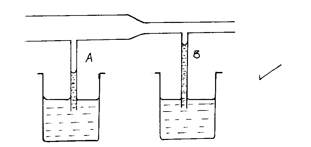3 marks

11.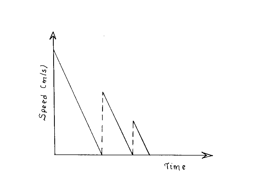3 marks

12.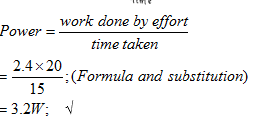2 marks

13.

It turns in anticlockwise direction expansion of air pushes the cork to the left hence shifting the centre of gravity of the tube to the left.

2 marks

14.

The block sinks further/submerged.

1 marks

### SECTION B (55 Marks)

Answer all the questions in this section in the spaces provided.
15.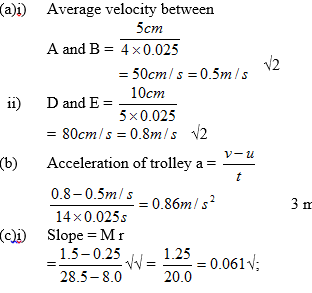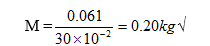ii) C is the T axis intercept C = -0.2N;; (Extraporation)

iii) Friction between the body and table

11 marks

16.

(a)i) Cooling is very fast initially from 1 to 2 sec then slows down.

-Cooling stops for 1 minute at 560C as naphthalene solidifies

. -Cooling continues for 6 seconds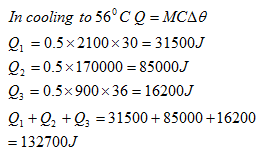Conductivity of aluminium can

Temperature of the surrounding air Any 2 (2 marks)

Surface area of aluminium can

)i) Gas pressure = atmospheric pressure = 760mmHg

ii)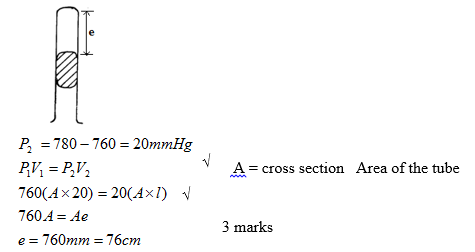12 marks

17.

(a) Force is the rate of change of momentum;

Momentum before collision=momentum after collision;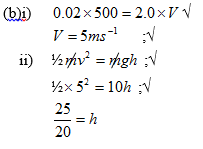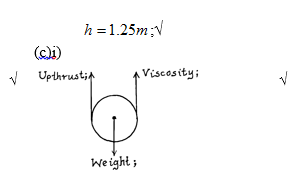11 marks

18.

(a) When a body is partially or totally immersed in a fluid, it experiences an upthrust Equal to weight of the fluid displaced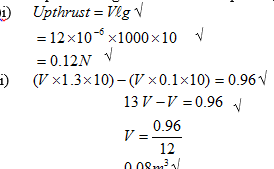(c)i A represents 0.8 while B represents 1.5; because for a denser liquid hydrometer sinks less while for less dense liquid it sinks more;

ii) To lower cog of hydrometer so that it float upright;

To displace more liquid so as to have sufficient upthrust

10 marks

19.

(a) Work is the product of force by the distance moved.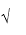SI unit = NM/Joule(b) Effect of weight of second pulley reduces efficiency of A or some of the effort applied in A goes to lifting the second pulley hence reduce efficiency of A; Load in B is larger and so effect of friction is less in B increasing efficiency but in A there greater friction due to the second pulley thus some effort goes to over the extra friction.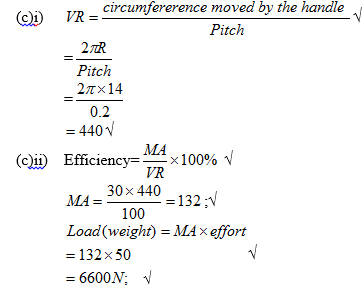9 marks

Back Top